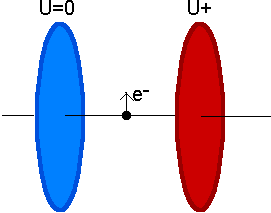QuickField

A new approach to field modelling
 Language: Global English Deutch Espanol Francais Italiano Danmark Ceske Chinese Pycckuü

order

evaluation

editions

version history

packages

components

programming

consulting

industrial

educational

scientific

sample problems

success stories

customers

 webinars virtual classroom online courses customer login glossary quickfield help faq
 quickfield student edition user manual data libraries video free tools
 product news events blog publications subscription
 tera analysis info resellers contact us online chat about us

 Examples gallery Step-by-step tutorials Verification examples Programming examples Distributive examples

>> >>

# Charged particle trajectory in the uniform static electric field. Case: cylindrical.

Problem Type:
Axisymmetric problem of electrostatics.

Geometry:Given:
Relative permittivity of vacuum ε = 1;
Positive potential U+ = 20 V.
Charge (electron) q = -1.602e-19 C
Mass (electron) m = 9.109e-31 kg
Initial velocity vy = 500 000 m/s; vz = vφ = 0 m/s.
Emitter position (0; 0; 0).

Problem:
Calculate the charged particle trajectory neglecting relativistic effects.

Solution:
The analytical solution is a parabola
x(t) = 0,
y(t) = vy * t,
z(t) = 0.5 * Fz / m * t2,
where Fz - is the z-component of the Lorentz force,
t - is time.

Particle trajectory may be calculated using the built-in function in QuickField Electrostatic postprocessor or by the free tool TrajectoryTracer.

Results:
Electric field strength Ez = -20 V/m, Er = 0 V/m

Lorentz force Fz = q * Ez = -1.602e-19 * -20 = 3.204e-18 N. x(t) = 0 m,
y(t) = 500000*t m,
z(t) = 0.5*3.204e-18/9.109e-31 *t2 m.

 Particle coordinates (x; y; z) m time Theory QuickField TrajectoryTracer tool 0 s (0; 0; 0) (0; 0; 0) (0; 0; 0) 1e-7 s (0; 0.050; 0.018) (0; 0.050; 0.018) (0; 0.050; 0.018) 2e-7 s (0; 0.100; 0.070) (0; 0.100; 0.070) (0; 0.100; 0.070) 3e-7 s (0; 0.150; 0.158) (0; 0.150; 0.158) (0; 0.150; 0.158) 4e-7 s (0; 0.200; 0.281) (0; 0.200; 0.281) (0; 0.200; 0.281) 5e-7 s (0; 0.250; 0.440) (0; 0.250; 0.440) (0; 0.250; 0.440)Watch on YouTube.Download simulation files (files may be viewed using any QuickField Edition).